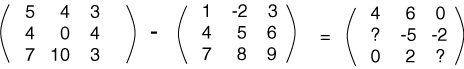It seems like subtraction could be defined as adding a negation of a matrix.

# Matrix Subtraction

If A and B have the same number of rows and columns, then:

A - B is defined as A + (-B).

Usually you think of this as:

To compute A - B, subtract the corresponding element of B from each element of A.

Here is a partly finished example:Notice in particular the elements in the first row of the answer. The way the result was calculated for the elements in row 1 column 2 is sometimes confusing.

### QUESTION 13:

Mentally fill in the two question marks.